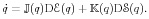WIAS Preprint No. 1943, (2014)

# On thermodynamical couplings of quantum mechanics and macroscopic systems

Authors

• Mielke, Alexander
ORCID: 0000-0002-4583-3888

2010 Mathematics Subject Classification

• 37N20 47N50 80A99 81V70 78A35

Keywords

• GENERIC, density matrix, Hamiltonian systems, Onsager systems, canonical correlation, heat reservoirs, non-equilibrium steady states, Maxwell-Bloch equation, thermo-opto-electronics

DOI

10.20347/WIAS.PREPRINT.1943

Abstract

Pure quantum mechanics can be formulated as a Hamiltonian system in terms of the Liouville equation for the density matrix. Dissipative effects are modeled via coupling to a macroscopic system, where the coupling operators act via commutators. Following Öttinger (2010) we use the GENERIC framework to construct thermodynamically consistent evolution equations as a sum of a Hamiltonian and a gradient-flow contribution, which satisfy a particular non-interaction condition:We give three applications of the theory. First, we consider a finite-dimensional quantum system that is coupled to a finite number of simple heat baths, each of which is described by a scalar temperature variable. Second, we model quantum system given by a one-dimensional Schrödinger operator connected to a one-dimensional heat equation on the left and on the right. Finally, we consider thermo-opto-electronics, where the Maxwell-Bloch system of optics is coupled to the energy-drift-diffusion system for semiconductor electronics.

Appeared in

• Mathematical Results in Quantum Mechanics. Proceedings of the QMath12 Conference, P. Exner, W. König, H. Neidhardt, eds., World Scientific Publishing, Singapore, 2015, pp. 331--348